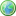Accessibility options:

# Vectors resources

Show me all resources applicable to

### Teach Yourself (4)Cartesian components of vectors
Any vector may be expressed in Cartesian components, by using unit vectors in the directions of the coordinate axes. In this unit we describe these unit vectors in two dimensions and in three dimensions, and show how they can be used in calculations.Introduction to vectors
A vector is a quantity that has both a magnitude (or size) and a direction. Both of these properties must be given in order to specify a vector completely. In this unit we describe how to write down vectors, how to add and subtract them, and how to use them in geometry.The scalar product
One of the ways in which two vectors can be combined is known as the scalar product. When we calculate the scalar product of two vectors the result, as the name suggests is a scalar, rather than a vector.The vector product
One of the ways in which two vectors can be combined is known as the vector product. When we calculate the vector product of two vectors the result, as the name suggests, is a vector.

### Test Yourself (1)Maths EG
Computer-aided assessment of maths, stats and numeracy from GCSE to undergraduate level 2. These resources have been made available under a Creative Common licence by Martin Greenhow and Abdulrahman Kamavi, Brunel University.

### Video (4)Cartesian components of vectors
Any vector may be expressed in Cartesian components, by using unit vectors in the directions of the coordinate axes. In this unit we describe these unit vectors in two dimensions and in three dimensions, and show how they can be used in calculations. (Mathtutor Video Tutorial). This resource is released under a Creative Commons license Attribution-Non-Commercial-No Derivative Works and the copyright is held by Skillbank Solutions Ltd.Introduction to vectors
A vector is a quantity that has both a magnitude (or size) and a direction. Both of these properties must be given in order to specify a vector completely. In this unit we describe how to write down vectors, how to add and subtract them, and how to use them in geometry. (Mathtutor Video Tutorial) This resource is released under a Creative Commons license Attribution-Non-Commercial-No Derivative Works and the copyright is held by Skillbank Solutions Ltd.The scalar product
One of the ways in which two vectors can be combined is known as the scalar product. When we calculate the scalar product of two vectors the result, as the name suggests is a scalar, rather than a vector. (Mathtutor Video Tutorial) This resource is released under a Creative Commons license Attribution-Non-Commercial-No Derivative Works and the copyright is held by Skillbank Solutions Ltd.The vector product
One of the ways in which two vectors can be combined is known as the vector product. When we calculate the vector product of two vectors the result, as the name suggests, is a vector. (Mathtutor Video Tutorial) This resource is released under a Creative Commons license Attribution-Non-Commercial-No Derivative Works and the copyright is held by Skillbank Solutions Ltd.

### Video with captions which require edits (4)Cartesian components of vectors
Any vector may be expressed in Cartesian components, by using unit vectors in the directions of the coordinate axes. In this unit we describe these unit vectors in two dimensions and in three dimensions, and show how they can be used in calculations. (Mathtutor Video Tutorial). The video is released under a Creative Commons license Attribution-Non-Commercial-No Derivative Works and the copyright is held by Skillbank Solutions Ltd.Introduction to vectors
A vector is a quantity that has both a magnitude (or size) and a direction. Both of these properties must be given in order to specify a vector completely. In this unit we describe how to write down vectors, how to add and subtract them, and how to use them in geometry. (Mathtutor Video Tutorial) The video is released under a Creative Commons license Attribution-Non-Commercial-No Derivative Works and the copyright is held by Skillbank Solutions Ltd.The scalar product
One of the ways in which two vectors can be combined is known as the scalar product. When we calculate the scalar product of two vectors the result, as the name suggests is a scalar, rather than a vector. (Mathtutor Video Tutorial) The video is released under a Creative Commons license Attribution-Non-Commercial-No Derivative Works and the copyright is held by Skillbank Solutions Ltd.The vector product
One of the ways in which two vectors can be combined is known as the vector product. When we calculate the vector product of two vectors the result, as the name suggests, is a vector. (Mathtutor Video Tutorial) The video is released under a Creative Commons license Attribution-Non-Commercial-No Derivative Works and the copyright is held by Skillbank Solutions Ltd.